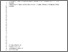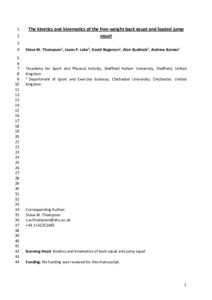# The kinetics and kinematics of the free-weight back squat and loaded jump squat

THOMPSON, Steve, LAKE, Jason, ROGERSON, David, RUDDOCK, Alan and BARNES, Andrew (2022). The kinetics and kinematics of the free-weight back squat and loaded jump squat. Journal of Strength and Conditioning Research.Preview
PDF
The kinetics and kinematics of the free-weight back squat and loaded jump squat.pdf - Accepted Version

## Abstract

The aim of this study was to compare kinetics and kinematics of 2 lower-body free-weight exercises, calculated from concentric and propulsion subphases, across multiple loads. Sixteen strength-trained men performed back squat 1 repetition maximum (1RM) tests (visit 1), followed by 2 incremental back squat and jump squat protocols (visit 2) (loads = 0% and 30–60%, back squat 1RM). Concentric phase and propulsion phase force-time-displacement characteristics were derived from force plate data and compared using analysis of variance and Hedges' g effect sizes. Intrasession reliability was calculated using intraclass correlation coefficient (ICC) and coefficient of variation (CV). All dependent variables met acceptable reliability (ICC >0.7; CV < 10%). Statistically significant 3-way interactions (load × phase × exercise) and 2-way main effects (phase × exercise) were observed for mean force, velocity (30–60% 1RM), power, work, displacement, and duration (0%, 30–50% 1RM) (p < 0.05). A significant 2-way interaction (load × exercise) was observed for impulse (p < 0.001). Jump squat velocity (g = 0.94–3.80), impulse (g = 1.98–3.21), power (g = 0.84–2.93), and work (g = 1.09–3.56) were significantly larger across concentric and propulsion phases, as well as mean propulsion force (g = 0.30–1.06) performed over all loads (p < 0.001). No statistically significant differences were observed for mean concentric force. Statistically longer durations (g = 0.38–1.54) and larger displacements (g = 2.03–4.40) were evident for all loads and both subphases (p < 0.05). Ballistic, lower-body exercise produces greater kinetic and kinematic outputs than nonballistic equivalents, irrespective of phase determination. Practitioners should therefore use ballistic methods when prescribing or testing lower-body exercises to maximize athlete's force-time-displacement characteristics.

Item Type: Article Sport Sciences; 1106 Human Movement and Sports Sciences; 1116 Medical Physiology https://doi.org/10.1519/JSC.0000000000004186 Symplectic Elements Symplectic Elements 03 Nov 2021 15:12 05 Jan 2023 01:18 https://shura.shu.ac.uk/id/eprint/29271View Item